[Next] [Up] [Previous]

# Computer Arithmetic

Because electronic logic deals with currents that are on or off, it has been found convenient to represent quantities in binary form to perform arithmetic on a computer. Thus, instead of having ten different digits, 0, 1, 2, 3, 4, 5, 6, 7, 8, and 9, in binary arithmetic, there are only two different digits, 0 and 1, and when moving to the next column, instead of the digit representing a quantity that is ten times as large, it only represents a quantity that is two times as large. Thus, the first few numbers are written in binary as follows:

```       Decimal   Binary
Zero        0        0
One         1        1
Two         2       10
Three       3       11
Four        4      100
Five        5      101
Six         6      110
Seven       7      111
Eight       8     1000
Nine        9     1001
Ten        10     1010
Eleven     11     1011
Twelve     12     1100
```

The addition and multiplication tables for binary arithmetic are very small, and this makes it possible to use logic circuits to build binary adders.

```+ | 0  1       * | 0  1
---------      ---------
0 | 0  1       0 | 0  0
1 | 1 10       1 | 0  1
```

Thus, from the table above, when two binary digits, A and B are added, the carry bit is simply (A AND B), while the last digit of the sum is more complicated; ((A AND NOT B) OR ((NOT A) AND B)) is one way to express it.

And multiplication becomes a series of shifts, accompanied by successive decisons of whether to add or not to add.

When a number like 127 is written in the decimal place-value system of notation, one can think of it as indicating that one has:

seven marbles,

two boxes that have ten marbles in each box,

one box that has ten boxes each with ten marbles in that box.

The binary system is another place-value system, based on the same principle; however, in that system, the smallest boxes only have two marbles in them, and any larger box contains only two of the next smaller size of box.

We have seen how we can use two digits, 0 and 1, to do the same thing that we can do with the digits 0 and 9 only; write integers equal to or greater than zero. In writing, it is easy enough to add a minus sign to the front of a number, or insert a decimal point. When a number is represented only as a string of bits, with no other symbols, special conventions must be adopted to represent a number that is negative, or one with a radix point indicating a binary fraction.

Historically, computers have represented negative numbers in several different ways.

One method is sign-magnitude representation, in which the first bit of the integer, if a one, indicates that the number is negative.

Another is one's complement notation, in which, when a number is negative, in addition to this being indicated by setting the first bit of the number to a one, the other bits of the number are all inverted.

By far the most common way to represent negative integers on modern computers, however, is two's complement notation, because in this notation, addition of signed quantities, except for the possible presence of a carry out when an overflow is not actually taking place, is identical to the normal addition of positive quantities. Here, to replace a number with its negative equivalent, one inverts all the bits, and then adds one.

Another method of representing negative numbers is simply to add a constant, equal to half the range of the integer type, to the number to obtain its binary representation. This is usually used for the exponent portion of floating-point numbers, as we will see later.

```Number  Binary representations                      Decimal representations

Sign-      One's      Two's      Excess-    Sign-     Nine's     Ten's      Excess-
magnitude  complement complement 512        magnitude complement complement 500

+511    0111111111 0111111111 0111111111 1111111111
+510    0111111110 0111111110 0111111110 1111111110
...
+500    0111110100 0111110100 0111110100 1111110100
+499    0111110011 0111110011 0111110011 1111110011 499       499        499        999
+498    0111110010 0111110010 0111110010 1111110010 498       498        498        998
...
+2      0000000010 0000000010 0000000010 1000000010 002       002        002        502
+1      0000000001 0000000001 0000000001 1000000001 001       001        001        501
0      0000000000 0000000000 0000000000 1000000000 000       000        000        500
-1      1000000001 1111111110 1111111111 0111111111 501       998        999        499
-2      1000000010 1111111101 1111111110 0111111110 502       997        998        498
...
-498    1111110010 1000001101 1000001110 0000001110 998       501        502        002
-499    1111110011 1000001100 1000001101 0000001101 999       500        501        001
-500    1111110100 1000001011 1000001100 0000001100                      500        000
...
-510    1111111110 1000000001 1000000010 0000000010
-511    1111111111 1000000000 1000000001 0000000001
-512                          1000000000 0000000000
```

Floating-point numbers are used in scientific calculations. In these calculations, instead of dealing in numbers which must always be exact in terms of a whole unit, whether that unit is a dollar or a cent, a certain number of digits of precision is sought for a quantity that might be very small or very large.

Thus, floating-point numbers are represented internally in a computer in something resembling scientific notation.

In scientific notation,

```                            6
1,230,000  becomes 1.23 * 10
```

and the common logarithm of 1,230,000 is 6.0899; the integer and fractional parts of a common logarithm are called, respectively, the characteristic and the mantissa when using common logarithms to perform calculations.

To keep the fields in a floating-point number distinct, and to make floating-point arithmetic simple, a common way to represent floating point numbers is like this:

First, the sign of the number; 0 if positive, 1 if negative.

Then the exponent. If the exponent is seven bits long, it is represented in excess-64 notation; if it is eleven bits long, as in this example, it is represented in excess-1,024 notation. That is, the bits used for the exponent are the binary representation of the exponent value plus 1,024; this is the simplest way to represent exponents, since everything is now positive.

Finally, the leading digits of the actual binary number. As these contain the same information as the fractional part of the base-2 logarithm (or the base-16 logarithm, had the exponent been a power of 16, as is the case on some other architectures instead of the one examined here) of the number, this part of a floating-point number is also called the mantissa, even if this term is much criticized as a misnomer.

The exponent is in excess 1,024 notation when the binary point of the mantissa is considered to precede its leading digit.

As the digits of the mantissa are not altered when the number is negative, sign-magnitude notation may be said to be what is used for that part of the number.

Some examples of floating-point numbers are shown below:

```1,024          0 10000001011 10000
512          0 10000001010 10000
256          0 10000001001 10000
128          0 10000001000 10000
64          0 10000000111 10000
32          0 10000000110 10000
16          0 10000000101 10000

14          0 10000000100 11100
12          0 10000000100 11000
10          0 10000000100 10100

8          0 10000000100 10000

7          0 10000000011 11100
6          0 10000000011 11000
5          0 10000000011 10100

4          0 10000000011 10000

3.5        0 10000000010 11100
3          0 10000000010 11000
2.5        0 10000000010 10100

2          0 10000000010 10000

1.75       0 10000000001 11100
1.5        0 10000000001 11000
1.25       0 10000000001 10100

1          0 10000000001 10000

0.875      0 10000000000 11100
0.75       0 10000000000 11000
0.625      0 10000000000 10100

0.5        0 10000000000 10000
0.25       0 01111111111 10000
0.125      0 01111111110 10000
0.0625     0 01111111101 10000
0.03125    0 01111111100 10000

0          0 00000000000 00000

-1          1 10000000001 10000
```

In each of the entries above, the entire 11-bit exponent field is shown, but only the first five bits of the mantissa field are shown, the rest being zero.

Note that for all the numbers except zero, the first bit of the mantissa is a one. This is somewhat wasteful; if the exponent is a power of 4, 8, or 16 instead of a power of two, then the restriction seen will only be that the first 2, 3, or 4 bits, respectively, of the mantissa will not all be zero.

A floating-point number whose first digit, where the size of a digit is determined by the base of the exponent, is not zero is said to be normalized. In general, when a floating-point number is normalized, it retains the maximum possible precision, and normalizing the result is an intrinsic part of any floating-point operation.

In the illustration above,

```               0 00000000000 10000
```

would represent the smallest number that can possibly be normalized. Some computers permit gradual underflow, where quantities such as

```               0 00000000000 01000
```

are also allowed, since they are as normalized as is possible, and their meaning is unambiguous.

### The Early Days of Hexadecimal

I don't know where else on my site to put this amusing bit of trivia.

Most computers, internally, use binary numbers for arithmetic, as circuits for binary arithmetic are the simplest to implement. Some computers will perform base-10 arithmetic instead, so as to directly calculate on numbers as they are used by people in writing. This is usually done by representing each digit in binary form, although a number of different codings for decimal digits have been used.

When a computer uses binary arithmetic, it is desirable to have a short way to represent binary numbers. One way to do this is to use octal notation, where the digits from 0 to 7 represent three consecutive bits; thus, integers in base-8 representation can be quickly translated to integers in base-2 representation. Another way, more suited to computers with word lengths that are multiples of four digits, is hexadecimal notation.

Today, programmers are familiar with the use of the letters A through F to represent the values 10 through 15 as single hexadecimal digits. Before the IBM System/360 made this the universal standard, some early computers used alternate notations for hexadecimal:

```                           10 11 12 13 14 15
System/360                  A  B  C  D  E  F
C                           a  b  c  d  e  f
Bendix G-15, SWAC           u  v  w  x  y  z
Monrobot XI                 S  T  U  V  W  X
Datamatic D-1000            b  c  d  e  f  g
Elbit 100                   B  C  D  E  F  G
LGP-30                      f  g  j  k  q  w
ILLIAC                      k  s  n  j  f  l
Pacific Data Systems 1020   L  C  A  S  M  D
```

And for some even more interesting trivia, if one goes from base-10 not to base-16, but only to base-12, while adding either the letters A and B, or the letters X and Y (what with X being the Roman numeral for ten), suggest themselves as alternatives, making new symbols is also conceivable.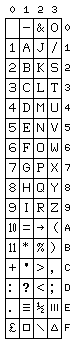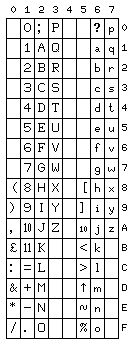On the right is pictured the character set for the LEO III computer, which placed 10 and 11 on single characters for use in printing amounts in pence with sterling currency. On the left, the character set of the Elliott 503 is pictured, exclusive of control characters; in addition to the 10 and 11 (at x'1A' and x'1B' respectively) following the digits 0 through 9 used for that purpose, the character set also contains a reduced-height 10 at x'5A': this character was used in ALGOL for printing out numbers in scientific notation; it replaced the letter E as used in FORTRAN, having the meaning "times 10 to the power", and is present as the character U+23E8 in Unicode today. This character set was based on a British Standards Institute proposal from 1956; note how this character set resembles ASCII in several respects.

That sterling currency led to symbols for base-12 notation being included in a computer character code, and one important in the history of ASCII as well, may seem surprising. In connection with the discussion above of different ways to write hexadecimal numbers, I allowed myself a digression concerning such matters as base-12 arithmetic which I have now relocated to a page of its own.

Incidentally, while the Elliott 503 was compatible with the earlier Elliott 803 in terms of its instruction set, the software for the earlier machine made use of a form of 5-level code. This code, however, wasn't the widely-used one based on Murray code, but instead assigned the letters to the codes from 1 to 26 in order, in a manner similar to that used with ASCII. The digits, however, had odd parity associated with them in that code: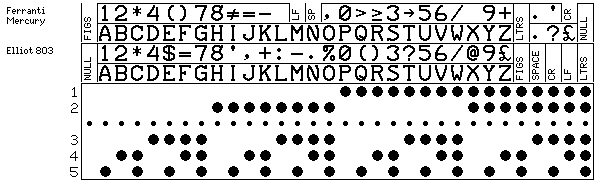The Ferranti Mercury computer, one of the earliest computers to use core memory, also had a 5-level code with the letters and digits in the same positions, but both the special characters and the control characters were different. While Figures Shift and Letters Shift needed to be always available, to allow for extra printable characters, Line Feed and Carriage Return were moved to be available in figures shift only.

Here is a side-by-side illustration of the code used in the Elliot 503, known as Cluff-Foster-Idelson code (although, according to the references I've seen, it was chiefly designed by the last named individual, one Ivan Idelson) and presented by Ferranti to the British Standards Institute, and ASCII, both shown as paper-tape codes, so as to illustrate the changed position of the parity bit.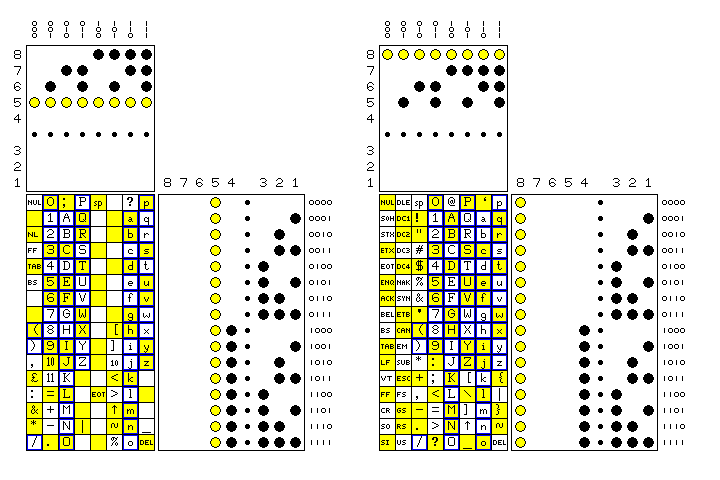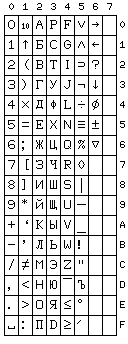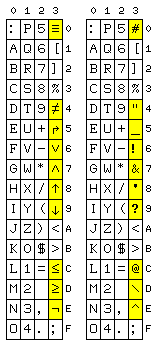Incidentally, these British codes are not the only ones to include some of the special characters defined for ALGOL 60. A character set that was more comprehensive in its inclusion of those characters was the GOST 10859-64 character set used in the Soviet Union, with the BESM-6 and Minsk-32 computers, for example, as depicted at right.

Note that the character at X'52' is used in Algol with the meaning 'implies' rather than what might be a more familiar meaning for a character of that shape, 'proper subset'.

Also, note that the characters from x'60' to x'66' were extra characters used on the Minsk-32, but not available on the BESM-6, and not, apparently, part of the GOST 10859-64 standard.

On the left, CDC Display Code is depicted. On the left of the diagram, the original form is shown, which has a number of the characters used in ALGOL. On the right is a modified form used later, as ALGOL became less popular, but ASCII became established as the standard character code for computers, which used the same characters as the 64-bit uppercase subset of ASCII.

This way, the software on CDC computers would not need to be converted to a different character code, but ASCII terminals could be used with them, and, with a little character translation, people would see the characters they expect on line printer output and so on.

Incidentally, this ASCII version of the CDC display code led to an amusing story. A famous computer scientist was so exclusively concerned with the abstract and academic aspects of the discipline, as opposed to the concrete details of how specific computers worked, that in the first edition of his book introducing a new computer language, he referred to the ASCII character set version of CDC display code simply as ASCII, as though it were ASCII proper.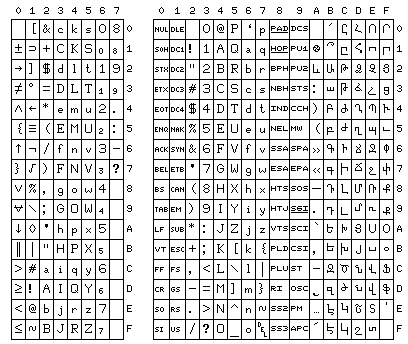And the character set for the STRETCH computer, officially known as the IBM 7030, is depicted above, on the left; this one also is clearly designed with ALGOL in mind. It had the unusual characteristic that the upper case and lower case letters were interleaved, in the hope that this would simplify collation. Another character set with this unusual characteristic is illustrated on the right, ARMSCII, an older modified version of ASCII to support the Armenian language.

Note that the STRETCH code has the alphabet in the order aAbBcC, while in the case of ARMSCII, while the Latin alphabet follows the normal ASCII sequence, the Armenian alphabet is in an order equivalent to AaBbCc.

Incidentally, ASCII has had quite an eventful history, as illustrated in the diagram below.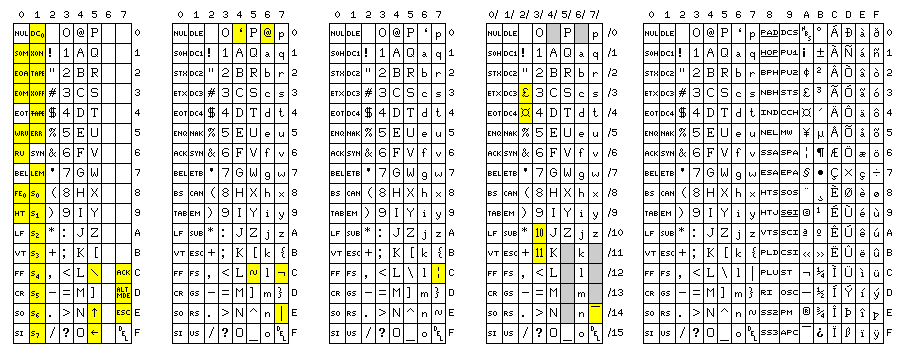This diagram shows five code tables. Code positions that differ from present-day ASCII are highlighted in yellow in each table.

The first table shows ASCII-1963, the original code used with such devices as the Model 33 Teletype. Many of the control characters had different names, but still had much the same function as they had later; thus, the "tab" character was originally called "horizontal tab".

The second table shows ASCII-1965, an ill-fated first attempt at adding lower-case to ASCII. For some obscure reason, the at-sign (@) was moved from its original position. Although it was voted into being as a standard, the standard was not officially published as a standard. None the less, it was not kept secret; it appeared, for example, in the September 1966 issue of Scientific American, a single topic issue about computers.

The third table shows the original form of ASCII-1967, complete with the broken vertical bar (since a solid vertical bar was an alternate representation of ! and the logical-not symbol was an alternate representation of ^; this had to do with allowing the uppercase-only subset of ASCII to better serve the programming language PL/I).

The fourth table shows the first version of ISO/IEC-646, the international version of ASCII. The sign for pound sterling, the international currency symbol, and the pence numbers for sterling currency are only possible alternates for #, \$, :, and ;, but the overline is the recommended form of ~. The tilde, as well as the character boxes in grey, are the standard national use positions. Note the use of the slash notation for code positions; this way, while a space can be ASCII code 40 (octal), 32 (decimal), or 20 (hex), it is unambiguously in code position 2/0.

The fifth table shows what we use today; officially, this character set is ISO 8859-1, and it is also the first 256 characters of Unicode, but it's often referred to as "8-bit ASCII".

After 1967, the National Bureau of Standards, now NIST, was renamed, and that led to the standard being renamed as well; in 1966, ASCII officially became USASCII, and in 1969 it officially became ANSCII, but these names were not generally used.

For completeness' sake, as a historical footnote, the diagram below is added: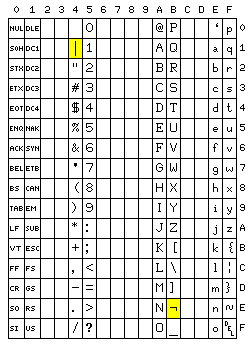This shows IBM's never-used USASCII-8, the version of ASCII the IBM System/360 was originally designed to be able to use. The bit in the Program Status Word which modified instructions like the EDIT instruction to convert integers into USASCII-8 characters instead of EBCDIC characters, as it was never used, ended up being repurposed in the IBM System/370 series to indicate Extended Control mode.

Note that the exclamation mark and the caret are replaced by vertical bar and logical NOT, so that PL/I programs could be expressed using only the upper-case subset of ASCII.

[Next] [Up] [Previous]